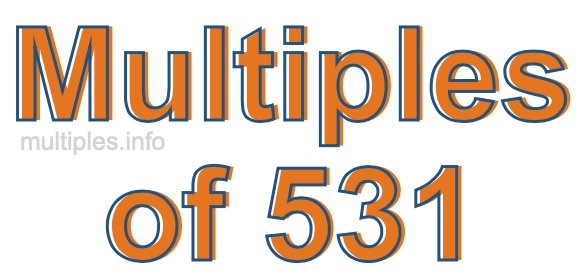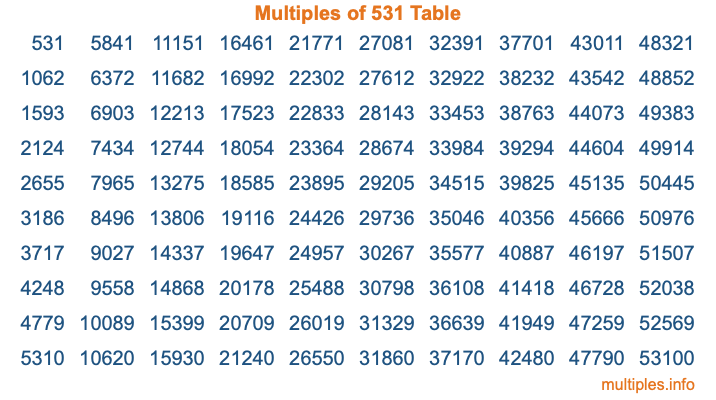Multiples of 531Welcome to the Multiples of 531 page. Here we will first teach you everything you will ever need to know about the multiples of 531, and then give you a study guide summary of everything we taught you to make sure you remember it all. Use this page to look up facts and learn information about the multiples of 531. This page will make you a multiples of five hundred thirty-one expert!

Definition of Multiples of 531
Multiples of 531 are all the numbers that when divided by 531 equal an integer. Each of the multiples of 531 are called a multiple. A multiple of 531 is created by multiplying 531 by an integer.

Therefore, to create a list of multiples of 531, you start with 1 multiplied by 531, then 2 multiplied by 531, then 3 multiplied by 531, and so on for as long as you want. Thus, the list of the first five multiples of 531 is 531, 1062, 1593, 2124, and 2655. To see a larger list of multiples of 531, see the printable image of Multiples of 531 further down on this page. We also have a category where you can choose any nth multiple of 531.

Multiples of 531 Checker
The Multiples of 531 Checker below checks to see if any number of your choice is a multiple of 531. In other words, it checks to see if there is any number (integer) that when multiplied by 531 will equal your number. To do that, we divide your number by 531. If the the quotient is an integer, then your number is a multiple of 531.

Is  a multiple of 531?

Least Common Multiple of 531 and ...
A Least Common Multiple (LCM) is the lowest multiple that two or more numbers have in common. This is also called the smallest common multiple or lowest common multiple and is useful to know when you are adding our subtracting fractions. Enter one or more numbers below (531 is already entered) to find the LCM.

Check out our LCM Calculator if you need more details about the Least Common Multiple or if you need the LCM for different numbers for adding and subtraction fractions.

nth Multiple of 531
As we stated above, 531 is the first multiple of 531, 1062 is the second multiple of 531, 1593 is the third multiple of 531, and so on. Enter a number below to find the nth multiple of 531.

th multiple of 531

Multiples of 531 vs Factors of 531
531 is a multiple of 531 and a factor of 531, but that is where the similarities end. All postive multiples of 531 are 531 or greater than 531. All positive factors of 531 are 531 or less than 531.

Below is the beginning list of multiples of 531 and the factors of 531 so you can compare:

Multiples of 531: 531, 1062, 1593, 2124, 2655, etc.

Factors of 531: 1, 3, 9, 59, 177, 531

As you can see, the multiples of 531 are all the numbers that you can divide by 531 to get a whole number. The factors of 531, on the other hand, are all the whole numbers that you can multiply by another whole number to get 531.

It's also interesting to note that if a number (x) is a factor of 531, then 531 will also be a multiple of that number (x).

Multiples of 531 vs Divisors of 531
The divisors of 531 are all the integers that 531 can be divided by evenly. Below is a list of the divisors of 531.

Divisors of 531: 1, 3, 9, 59, 177, 531

The interesting thing to note here is that if you take any multiple of 531 and divide it by a divisor of 531, you will see that the quotient is an integer.

Multiples of 531 Table
Below is an image of the first 100 multiples of 531 in a table. The table is in chronological order, column by column. The first column has the first ten multiples of 531, the second column has the next ten multiples of 531, and so on.The Multiples of 531 Table is also referred to as the 531 Times Table or Times Table of 531. You are welcome to print out our table for your studies.

Negative Multiples of 531
Although not often discussed or needed in math, it is worth mentioning that you can make a list of negative multiples of 531 by multiplying 531 by -1, then by -2, then by -3, and so on, to get the following list of negative multiples of 531:

-531, -1062, -1593, -2124, -2655, etc.

Multiples of 531 Summary
Below is a summary of important Multiples of 531 facts that we have discussed on this page. To retain the knowledge on this page, we recommend that you read through the summary and explain to yourself or a study partner why they hold true.

There are an infinite number of multiples of 531.

A multiple of 531 divided by 531 will equal a whole number.

531 divided by a factor of 531 equals a divisor of 531.

The nth multiple of 531 is n times 531.

The largest factor of 531 is equal to the first positive multiple of 531.

531 is a multiple of every factor of 531.

531 is a multiple of 531.

A multiple of 531 divided by a divisor of 531 equals an integer.

531 divided by a divisor of 531 equals a factor of 531.

Any integer times 531 will equal a multiple of 531.

Multiples of a Number
Here you can get the multiples of another number, all with the same attention to detail as we did for multiples of 531 on this page.

Multiples of
Multiples of 532
Did you find our page about multiples of five hundred thirty-one educational? Do you want more knowledge? Check out the multiples of the next number on our list!